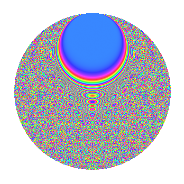# Properties

 Label 525.4.bmLevel $525$ Weight $4$ Character orbit 525.bm Rep. character $\chi_{525}(131,\cdot)$ Character field $\Q(\zeta_{30})$ Dimension $1888$ Sturm bound $320$

# Related objects

## Defining parameters

 Level: $$N$$ $$=$$ $$525 = 3 \cdot 5^{2} \cdot 7$$ Weight: $$k$$ $$=$$ $$4$$ Character orbit: $$[\chi]$$ $$=$$ 525.bm (of order $$30$$ and degree $$8$$) Character conductor: $$\operatorname{cond}(\chi)$$ $$=$$ $$525$$ Character field: $$\Q(\zeta_{30})$$ Sturm bound: $$320$$

## Dimensions

The following table gives the dimensions of various subspaces of $$M_{4}(525, [\chi])$$.

Total New Old
Modular forms 1952 1952 0
Cusp forms 1888 1888 0
Eisenstein series 64 64 0

## Trace form

 $$1888q - 9q^{3} - 934q^{4} - 20q^{7} - 3q^{9} + O(q^{10})$$ $$1888q - 9q^{3} - 934q^{4} - 20q^{7} - 3q^{9} - 60q^{10} + 39q^{12} - 506q^{15} + 3546q^{16} + 40q^{18} - 18q^{19} - 111q^{21} + 808q^{22} - 570q^{24} + 82q^{25} + 1484q^{28} + 677q^{30} - 1260q^{31} - 1449q^{33} + 344q^{36} - 222q^{37} - 649q^{39} - 2328q^{40} + 116q^{42} + 1280q^{43} + 2088q^{45} + 286q^{46} - 1028q^{49} + 472q^{51} - 786q^{52} + 39q^{54} + 2172q^{57} - 170q^{58} - 2947q^{60} - 18q^{61} - 455q^{63} + 27864q^{64} - 183q^{66} + 1002q^{67} + 4692q^{70} + 1089q^{72} - 1818q^{73} - 6102q^{75} - 4550q^{78} - 594q^{79} + 209q^{81} - 8628q^{82} + 1437q^{84} - 8548q^{85} + 3465q^{87} - 5298q^{88} + 5148q^{91} + 3812q^{93} + 270q^{94} - 4329q^{96} + 516q^{99} + O(q^{100})$$

## Decomposition of $$S_{4}^{\mathrm{new}}(525, [\chi])$$ into newform subspaces

The newforms in this space have not yet been added to the LMFDB.

## Hecke characteristic polynomials

There are no characteristic polynomials of Hecke operators in the database